Скачать презентацию 10 -6 Equations of Circles Warm Up Lesson

• Количество слайдов: 23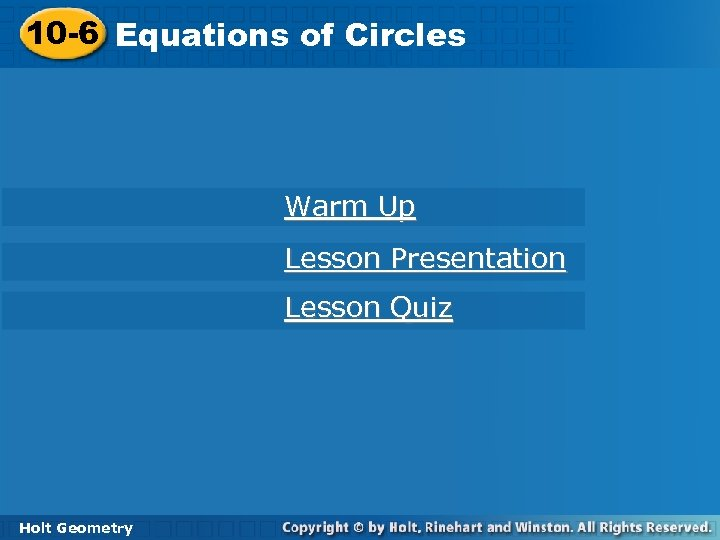10 -6 Equations of Circles Warm Up Lesson Presentation Lesson Quiz Holt Geometry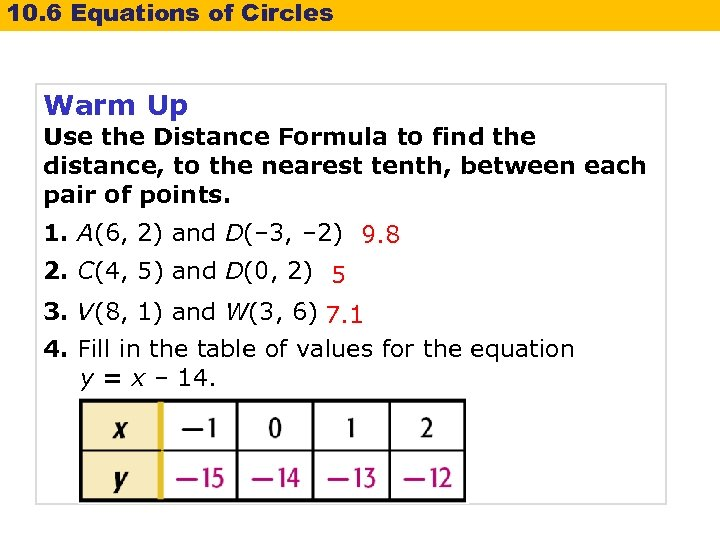10. 6 Equations of Circles Warm Up Use the Distance Formula to find the distance, to the nearest tenth, between each pair of points. 1. A(6, 2) and D(– 3, – 2) 9. 8 2. C(4, 5) and D(0, 2) 5 3. V(8, 1) and W(3, 6) 7. 1 4. Fill in the table of values for the equation y = x – 14.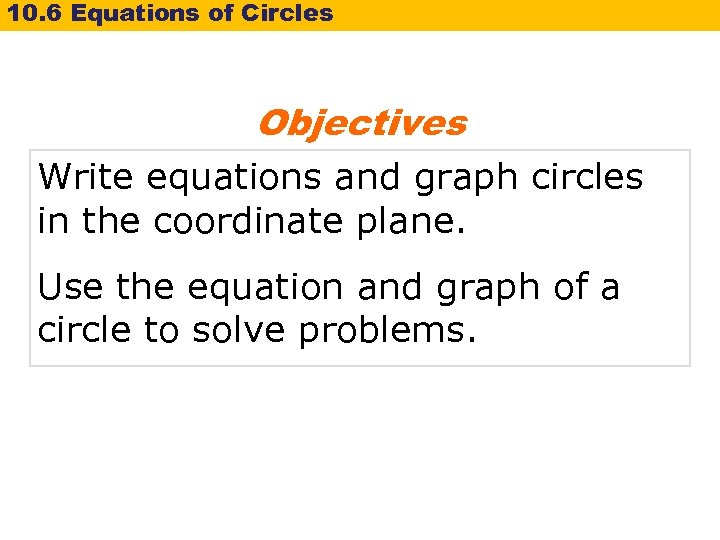10. 6 Equations of Circles Objectives Write equations and graph circles in the coordinate plane. Use the equation and graph of a circle to solve problems.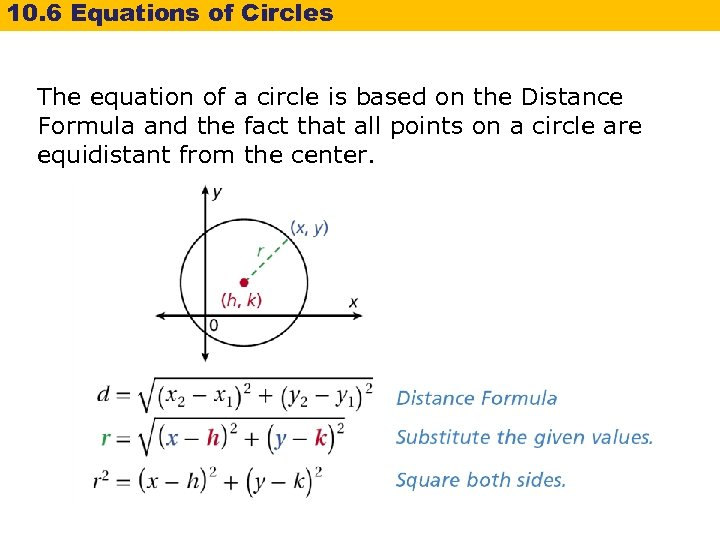10. 6 Equations of Circles The equation of a circle is based on the Distance Formula and the fact that all points on a circle are equidistant from the center.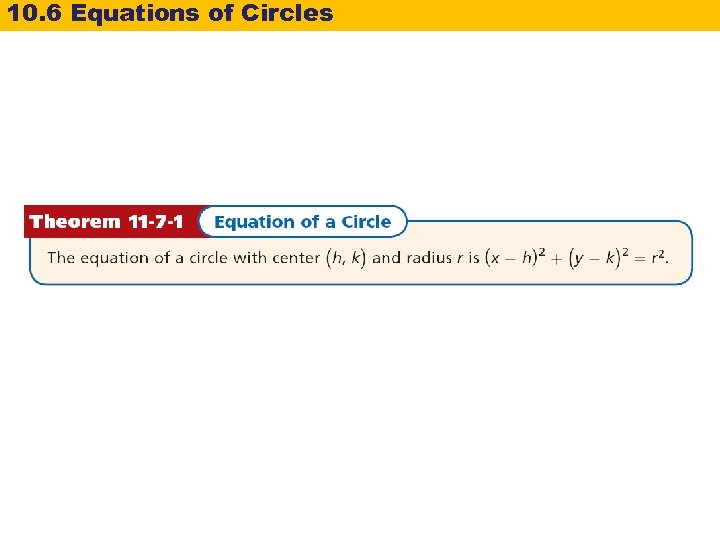10. 6 Equations of Circles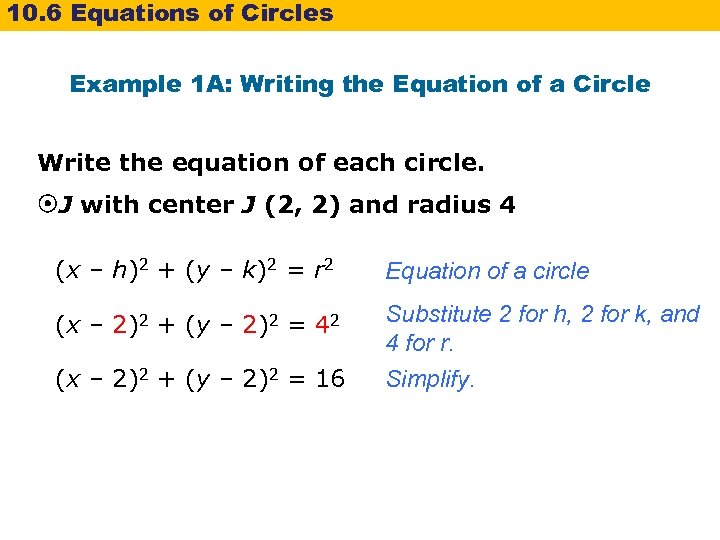10. 6 Equations of Circles Example 1 A: Writing the Equation of a Circle Write the equation of each circle. J with center J (2, 2) and radius 4 (x – h)2 + (y – k)2 = r 2 Equation of a circle (x – 2)2 + (y – 2)2 = 42 Substitute 2 for h, 2 for k, and 4 for r. Simplify. (x – 2)2 + (y – 2)2 = 16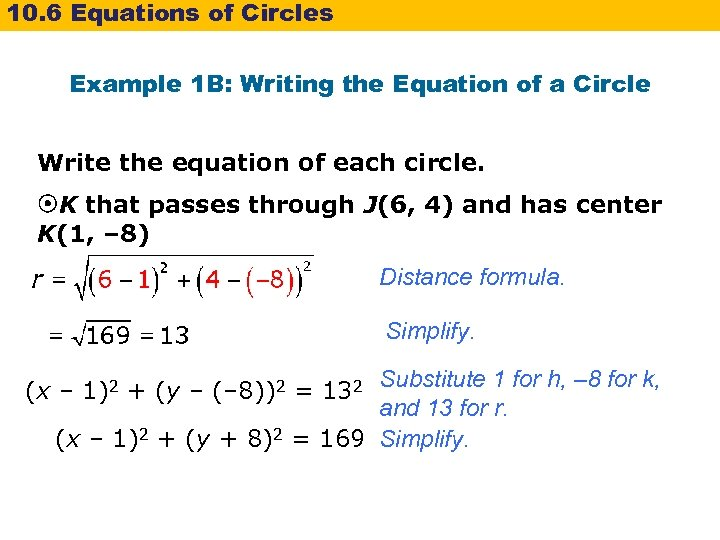10. 6 Equations of Circles Example 1 B: Writing the Equation of a Circle Write the equation of each circle. K that passes through J(6, 4) and has center K(1, – 8) Distance formula. Simplify. Substitute 1 for h, – 8 for k, and 13 for r. (x – 1)2 + (y + 8)2 = 169 Simplify. (x – 1)2 + (y – (– 8))2 = 132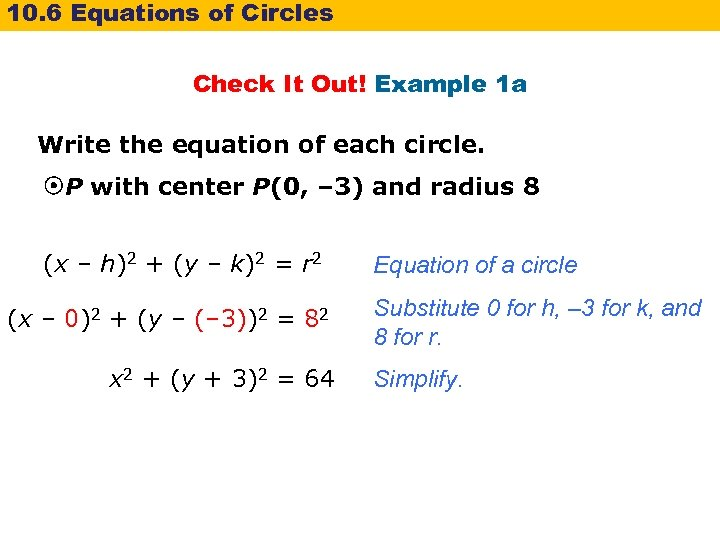10. 6 Equations of Circles Check It Out! Example 1 a Write the equation of each circle. P with center P(0, – 3) and radius 8 (x – h)2 + (y – k)2 = r 2 (x – 0)2 + (y – (– 3))2 = 82 x 2 + (y + 3)2 = 64 Equation of a circle Substitute 0 for h, – 3 for k, and 8 for r. Simplify.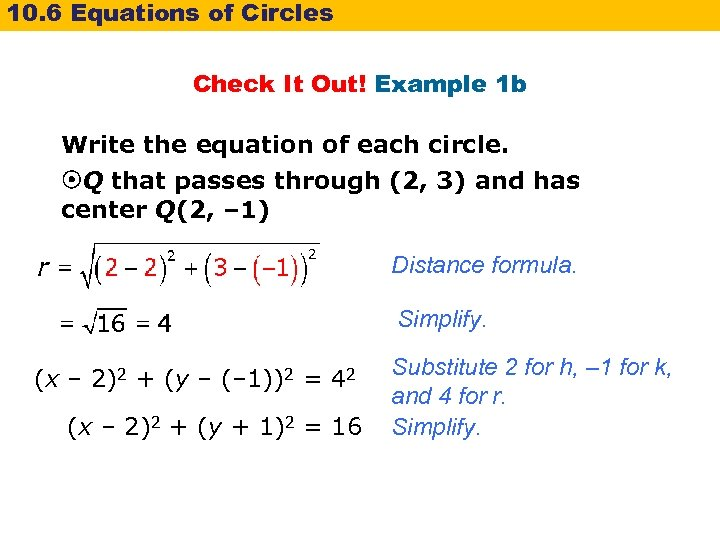10. 6 Equations of Circles Check It Out! Example 1 b Write the equation of each circle. Q that passes through (2, 3) and has center Q(2, – 1) Distance formula. Simplify. (x – 2)2 + (y – (– 1))2 = 42 (x – 2)2 + (y + 1)2 = 16 Substitute 2 for h, – 1 for k, and 4 for r. Simplify.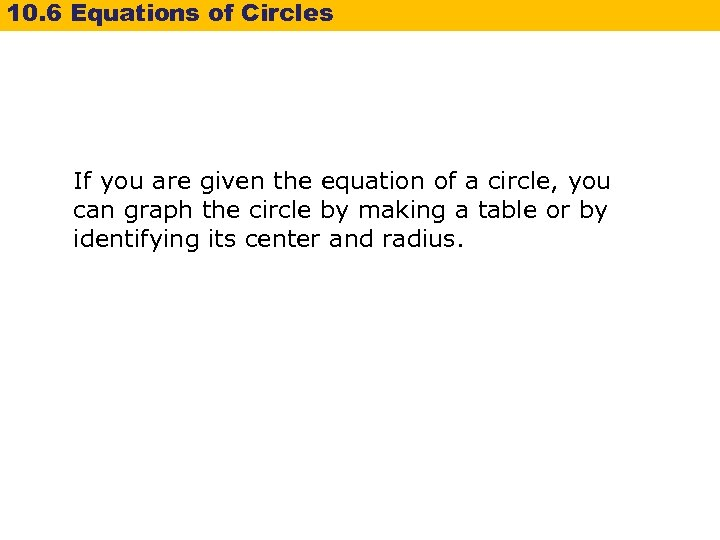10. 6 Equations of Circles If you are given the equation of a circle, you can graph the circle by making a table or by identifying its center and radius.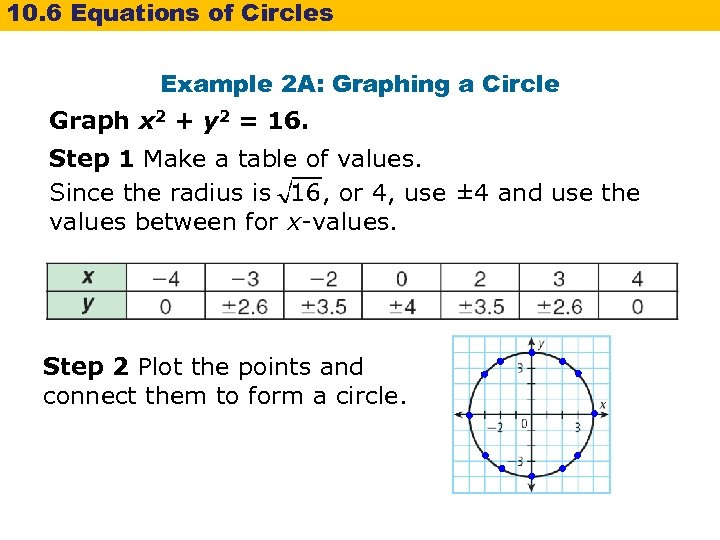10. 6 Equations of Circles Example 2 A: Graphing a Circle Graph x 2 + y 2 = 16. Step 1 Make a table of values. Since the radius is , or 4, use ± 4 and use the values between for x-values. Step 2 Plot the points and connect them to form a circle.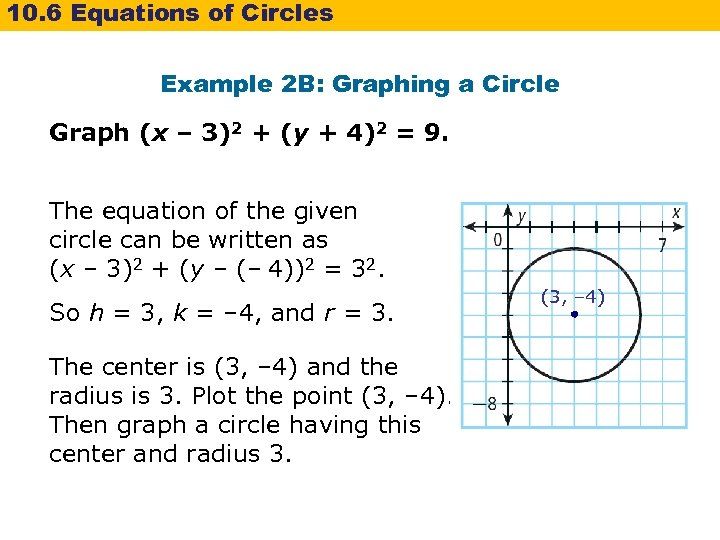10. 6 Equations of Circles Example 2 B: Graphing a Circle Graph (x – 3)2 + (y + 4)2 = 9. The equation of the given circle can be written as (x – 3)2 + (y – (– 4))2 = 32. So h = 3, k = – 4, and r = 3. The center is (3, – 4) and the radius is 3. Plot the point (3, – 4). Then graph a circle having this center and radius 3. (3, – 4)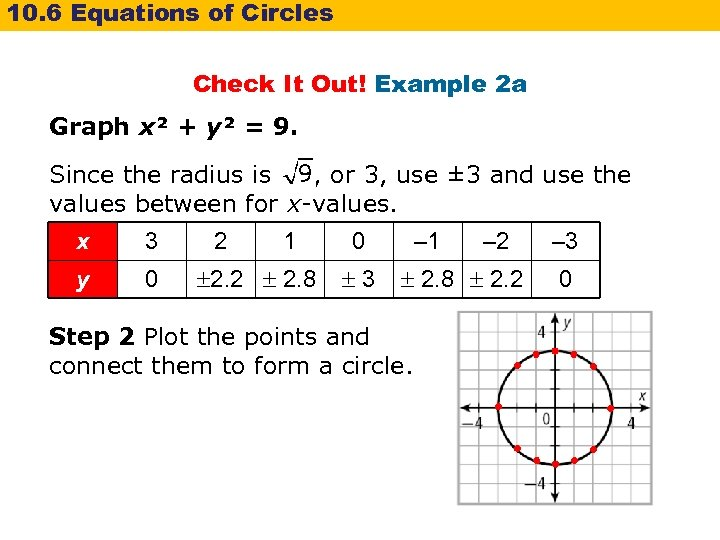10. 6 Equations of Circles Check It Out! Example 2 a Graph x² + y² = 9. Since the radius is , or 3, use ± 3 and use the values between for x-values. x 3 y 0 2 1 2. 2 2. 8 0 3 – 1 – 2 2. 8 2. 2 Step 2 Plot the points and connect them to form a circle. – 3 0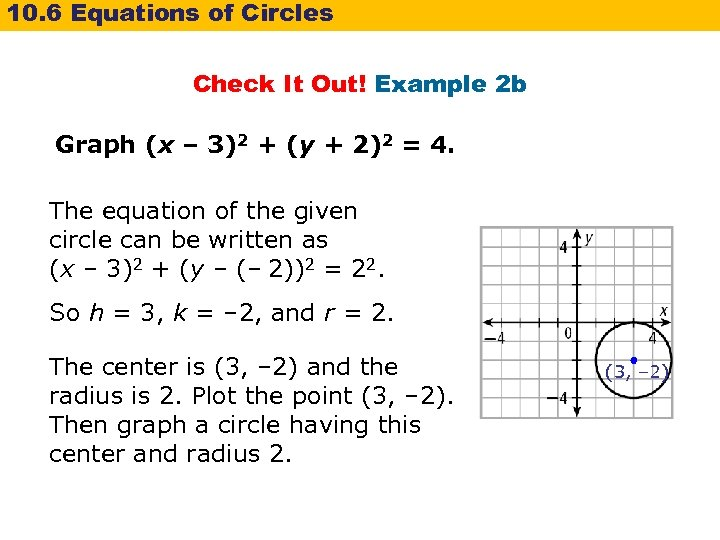10. 6 Equations of Circles Check It Out! Example 2 b Graph (x – 3)2 + (y + 2)2 = 4. The equation of the given circle can be written as (x – 3)2 + (y – (– 2))2 = 22. So h = 3, k = – 2, and r = 2. The center is (3, – 2) and the radius is 2. Plot the point (3, – 2). Then graph a circle having this center and radius 2. (3, – 2)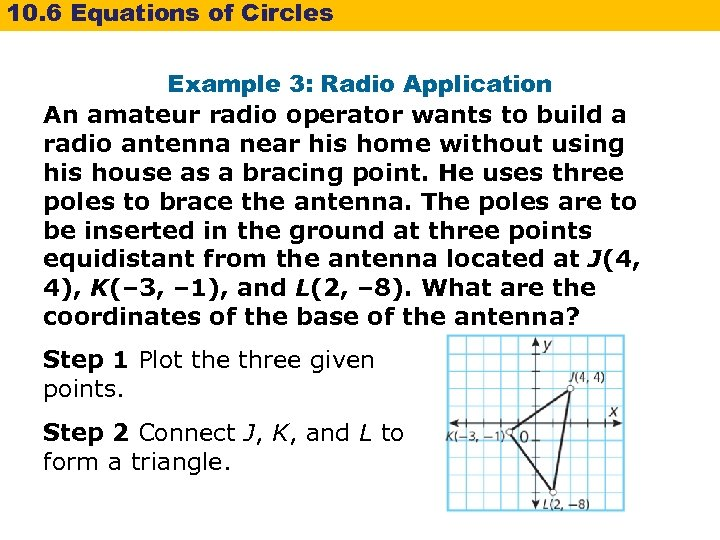10. 6 Equations of Circles Example 3: Radio Application An amateur radio operator wants to build a radio antenna near his home without using his house as a bracing point. He uses three poles to brace the antenna. The poles are to be inserted in the ground at three points equidistant from the antenna located at J(4, 4), K(– 3, – 1), and L(2, – 8). What are the coordinates of the base of the antenna? Step 1 Plot the three given points. Step 2 Connect J, K, and L to form a triangle.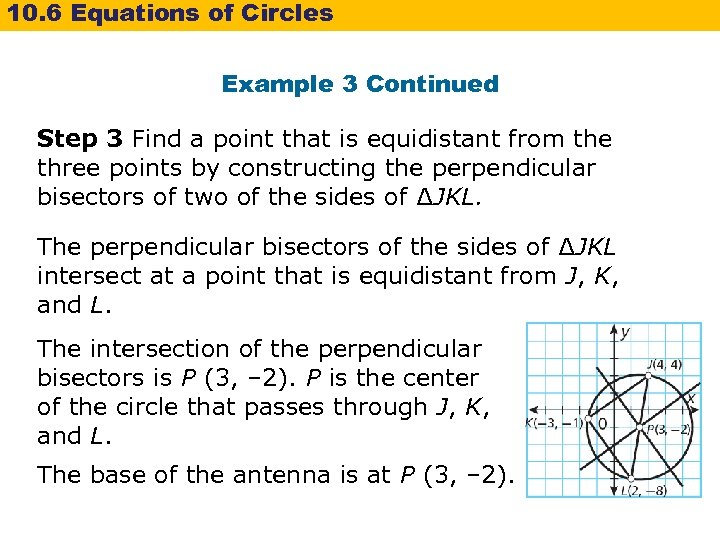10. 6 Equations of Circles Example 3 Continued Step 3 Find a point that is equidistant from the three points by constructing the perpendicular bisectors of two of the sides of ∆JKL. The perpendicular bisectors of the sides of ∆JKL intersect at a point that is equidistant from J, K, and L. The intersection of the perpendicular bisectors is P (3, – 2). P is the center of the circle that passes through J, K, and L. The base of the antenna is at P (3, – 2).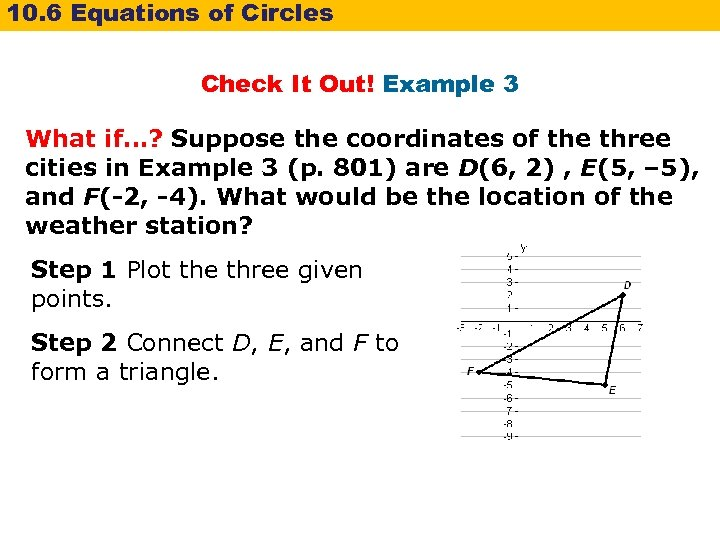10. 6 Equations of Circles Check It Out! Example 3 What if…? Suppose the coordinates of the three cities in Example 3 (p. 801) are D(6, 2) , E(5, – 5), and F(-2, -4). What would be the location of the weather station? Step 1 Plot the three given points. Step 2 Connect D, E, and F to form a triangle.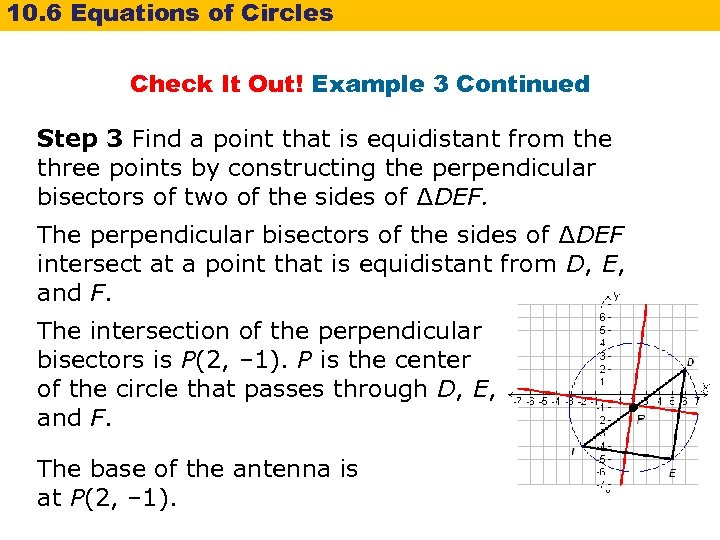10. 6 Equations of Circles Check It Out! Example 3 Continued Step 3 Find a point that is equidistant from the three points by constructing the perpendicular bisectors of two of the sides of ∆DEF. The perpendicular bisectors of the sides of ∆DEF intersect at a point that is equidistant from D, E, and F. The intersection of the perpendicular bisectors is P(2, – 1). P is the center of the circle that passes through D, E, and F. The base of the antenna is at P(2, – 1).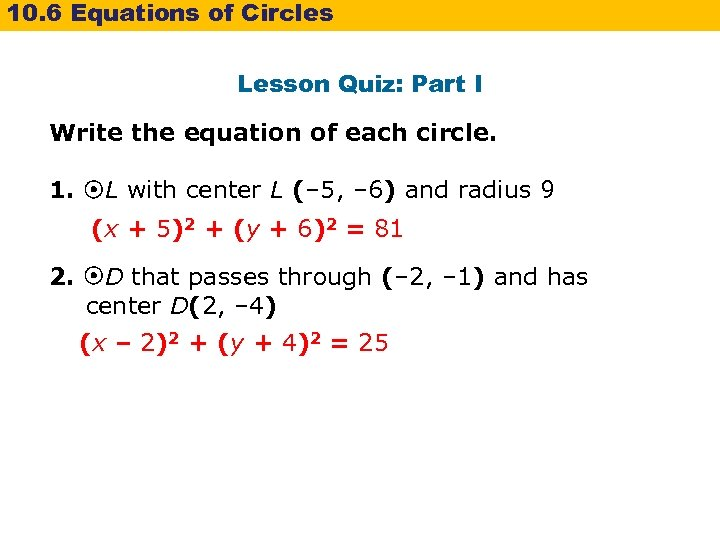10. 6 Equations of Circles Lesson Quiz: Part I Write the equation of each circle. 1. L with center L (– 5, – 6) and radius 9 (x + 5)2 + (y + 6)2 = 81 2. D that passes through (– 2, – 1) and has center D(2, – 4) (x – 2)2 + (y + 4)2 = 25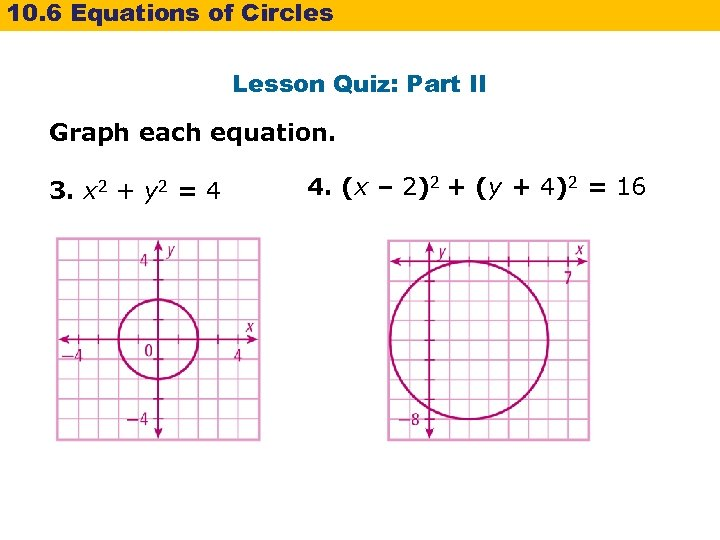10. 6 Equations of Circles Lesson Quiz: Part II Graph each equation. 3. x 2 + y 2 = 4 4. (x – 2)2 + (y + 4)2 = 16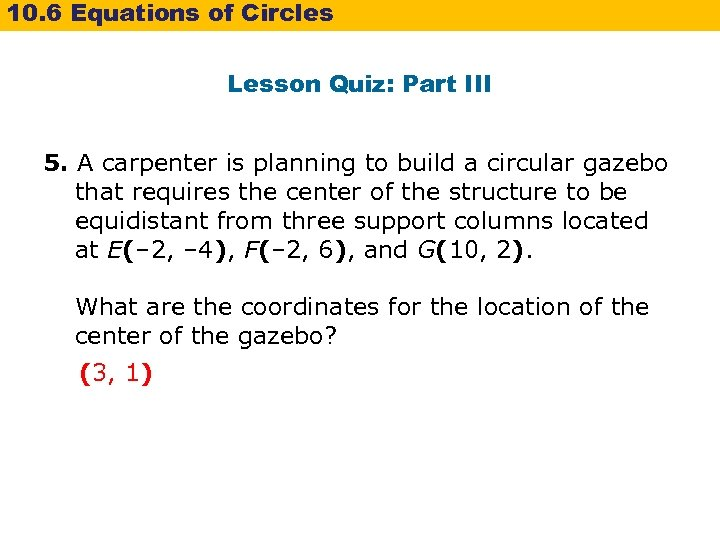10. 6 Equations of Circles Lesson Quiz: Part III 5. A carpenter is planning to build a circular gazebo that requires the center of the structure to be equidistant from three support columns located at E(– 2, – 4), F(– 2, 6), and G(10, 2). What are the coordinates for the location of the center of the gazebo? (3, 1)10. 6 Equations of Circles Lesson Quiz: Part III 5. A carpenter is planning to build a circular gazebo that requires the center of the structure to be equidistant from three support columns located at E(– 2, – 4), F(– 2, 6), and G(10, 2). What are the coordinates for the location of the center of the gazebo? (3, 1)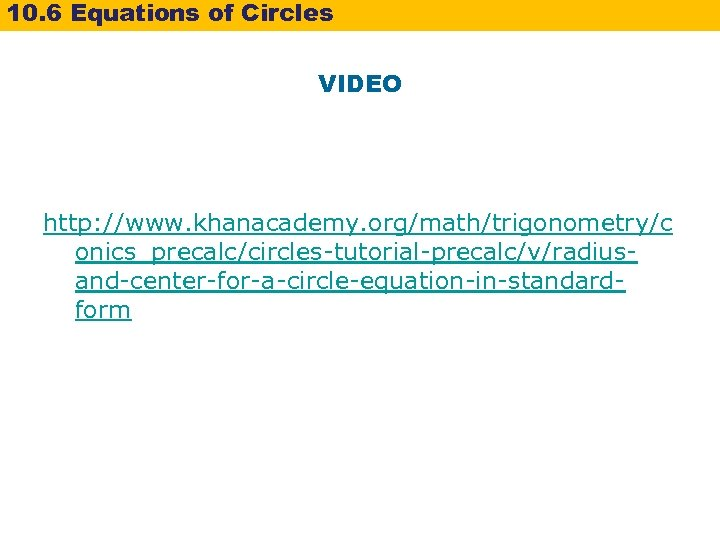10. 6 Equations of Circles VIDEO http: //www. khanacademy. org/math/trigonometry/c onics_precalc/circles-tutorial-precalc/v/radiusand-center-for-a-circle-equation-in-standardform